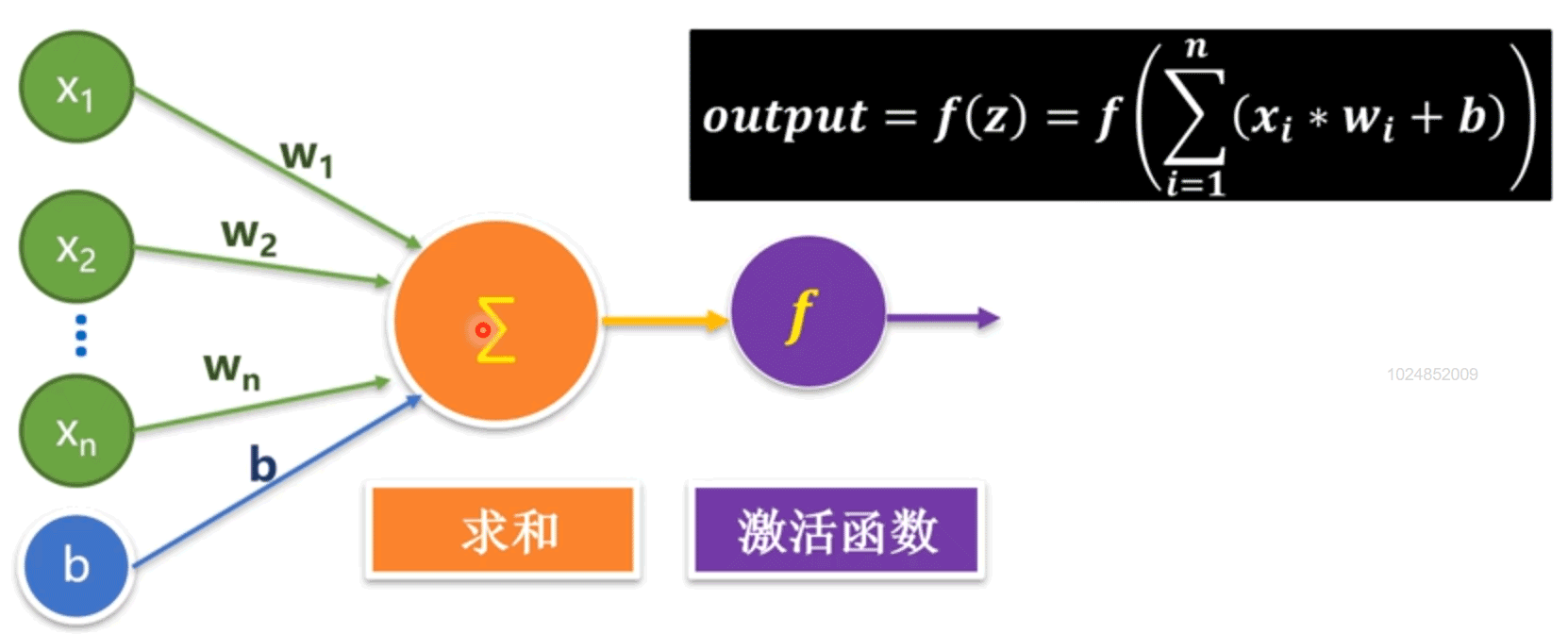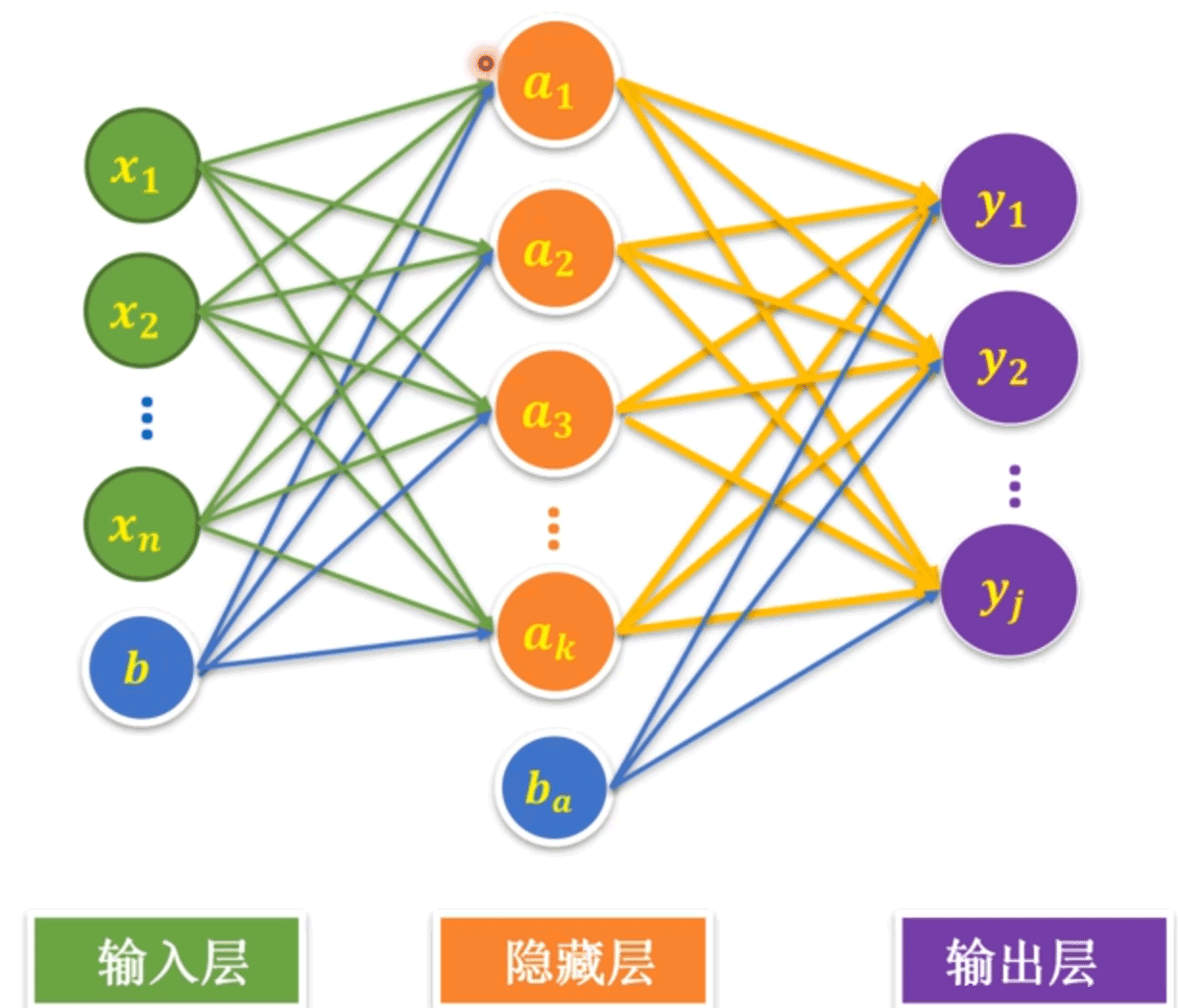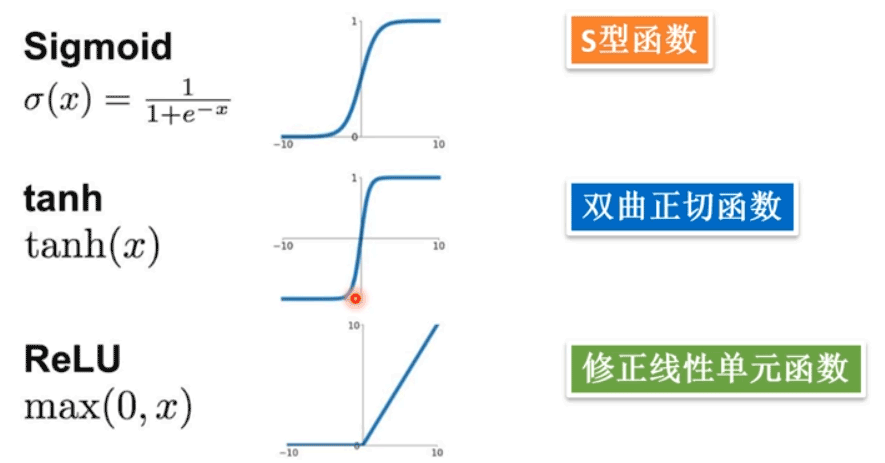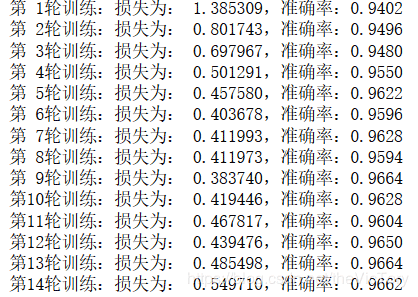# Python利用全连接神经网络求解MNIST问题详解

更新时间：2020年01月14日 11:35:42   作者：theVicTory我要评论

## 1、单隐藏层神经网络```import tensorflow as tf
import tensorflow.examples.tutorials.mnist.input_data as input_data

```x=tf.placeholder(tf.float32,[None,784],name='x')
y=tf.placeholder(tf.float32,[None,10],name='y')```

```h1_num=256                        #设置隐藏层神经元数量
W1=tf.Variable(tf.random_normal([784,h1_num]),name='W1')
b1=tf.Variable(tf.zeros([h1_num]),name='b1')
Y1=tf.nn.relu(tf.matmul(x,W1)+b1)             #激活函数```

```W2=tf.Variable(tf.random_normal([h1_num,10]),name='W2')
b2=tf.Variable(tf.zeros(),name='b2')
Y2=tf.matmul(Y1,W2)+b2
pred=tf.nn.softmax(Y2)```

```train_epochs=20                    #训练轮数
batch_size=50                     #每个批次的样本数
batch_num=int(mnist.train.num_examples/batch_size)  #一轮需要训练多少批
learning_rate=0.01
#定义损失函数、优化器
loss_function=tf.reduce_mean(             #softmax交叉熵损失函数
tf.nn.softmax_cross_entropy_with_logits(logits=Y2,labels=y))
#定义准确率
correct_prediction=tf.equal(tf.argmax(pred,1),tf.argmax(y,1))
accuracy=tf.reduce_mean(tf.cast(correct_prediction,tf.float32))```

```ss=tf.Session()
ss.run(tf.global_variables_initializer())           #进行全部变量的初始化

for epoch in range(train_epochs):
for batch in range(batch_num):              #分批次读取数据进行训练
xs,ys=mnist.train.next_batch(batch_size)
ss.run(optimizer,feed_dict={x:xs,y:ys})
loss,acc=ss.run([loss_function,accuracy],\
feed_dict={x:mnist.validation.images,y:mnist.validation.labels})
print('第%2d轮训练：损失为：%9f，准确率：%.4f'%(epoch+1,loss,acc))

ss.close()``````test_res=ss.run(accuracy,feed_dict={x:mnist.test.images,y:mnist.test.labels})
print('测试集的准确率为：%.4f'%(test_res))```

## 2、多层神经网络

```#构建输入层
x=tf.placeholder(tf.float32,[None,784],name='x')
y=tf.placeholder(tf.float32,[None,10],name='y')
#构建第一个隐藏层
h1_num=256                            #第一隐藏层神经元数量256
W1=tf.Variable(tf.truncated_normal([784,h1_num],stddev=0.1),name='W1')
b1=tf.Variable(tf.zeros([h1_num]),name='b1')
Y1=tf.nn.relu(tf.matmul(x,W1)+b1)
#构建第二个隐藏层
h2_num=64                             #第二隐藏层神经元数量64
W2=tf.Variable(tf.random_normal([h1_num,h2_num],stddev=0.1),name='W2')
b2=tf.Variable(tf.zeros([h2_num]),name='b2')
Y2=tf.nn.relu(tf.matmul(Y1,W2)+b2)
#构建输出层
W3=tf.Variable(tf.random_normal([h2_num,10],stddev=0.1),name='W3')
b3=tf.Variable(tf.zeros(),name='b3')
Y3=tf.matmul(Y2,W3)+b3
pred=tf.nn.softmax(Y3)```

### 全连接层函数

```#定义一个通用的全连接层函数模型
def fcn_layer(inputs,in_dim,out_dim,activation=None):
W=tf.Variable(tf.truncated_normal([in_dim,out_dim],stddev=0.1))
b=tf.Variable(tf.zeros([out_dim]))
Y=tf.matmul(inputs,W)+b
if activation==None:
output=Y
else:
output=activation(Y)
return output
#构建第一个隐藏层
Y1=fcn_layer(x,784,256,tf.nn.relu)
#构建第二个隐藏层
Y2=fcn_layer(Y1,256,64,tf.nn.relu)
#构建输出层
Y3=fcn_layer(Y2,64,10)
pred=tf.nn.softmax(Y3)```

## 3、模型的保存与读取

### 模型的储存

```import os
save_dir='D:/Temp/MachineLearning/ModelSaving/'    #定义模型的保存路径
if not os.path.exists(save_dir):            #如果不存在该路径则创建
os.makedirs(save_dir)```

```save_step=5            #定义存储粒度

saver=tf.train.Saver()      #定义saver```

```if epoch%save_step==0:
saver.save(ss,os.path.join(save_dir,'mnist_fcn_{:02d}.ckpt'.format(epoch+1)))```

`saver.save(ss,os.path.join(save_dir,'mnist_fcn.ckpt'))`

### 模型的读取

```save_dir='D:/Temp/MachineLearning/ModelSaving/'    #定义模型的保存路径
saver=tf.train.Saver()                 #定义saver

ss=tf.Session()
ss.run(tf.global_variables_initializer())

ckpt=tf.train.get_checkpoint_state(save_dir)      #读取存盘点
if ckpt and ckpt.model_checkpoint_path:
saver.restore(ss,ckpt.model_checkpoint_path)    #从存盘中恢复参数到当前的session
print('数据恢复从',ckpt.model_checkpoint_path)

test_res=accuracy.eval(session=ss,feed_dict={x:mnist.test.images,y:mnist.test.labels})
print('测试集的准确率为：%.4f'%(test_res))```

`NotFoundError (see above for traceback): Restoring from checkpoint failed. This is most likely due to a Variable name or other graph key that is missing from the checkpoint. Please ensure that you have not altered the graph expected based on the checkpoint.`

### 通过图来保存模型

```import tensorflow as tf

v=tf.Variable(1.0,'new_var')
with tf.Session() as ss:
tf.train.write_graph(ss.graph_def,'D:\Temp\MachineLearning\ModelSaving\Graph',
'test_graph.pb',as_text=False)```

```with tf.Session() as ss:
with tf.gfile.GFile('D:/Temp\MachineLearning/ModelSaving/Graph/test_graph.pb','rb') as pb_file:
graph_def=tf.GraphDef()